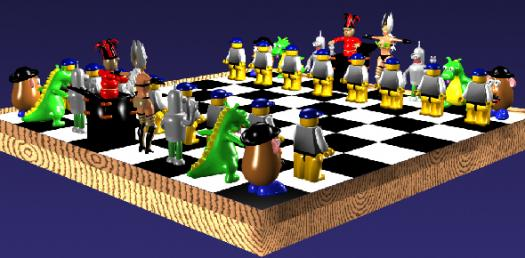# Computer Graphics For Animation Final Study Quiz

91 Questions | Total Attempts: 118SettingsFinal exam for Introduction to Computer Graphics for Animation

Related Topics
• 1.
Software written by a company for exclusive use within the company and not for sale to the general public is called:
• A.

Self proprietary

• B.

Non-proprietary

• C.

Proprietary

• 2.
Which of the following operating systems can be legally used free of charge?
• A.

OSX

• B.

Linux

• C.

Windows

• D.

Unix

• E.

None of the above

• 3.
Which of the following is not a "flavor" of UNIX?
• A.

Windows NT

• B.

Linux

• C.

Mac OSX

• D.

None of the above

• 4.
Match the unit of measurement most appropriate to describe the computer in front of you:
• A.

Kilabyte

• B.

Megabyte

• C.

Gigabytes

• 5.
What does the acronym GUI stand for (for example, SCUBA stands for Self Contained Underwater Breathing Apparatus)?
• A.

GUI

• B.

Graphical User Interface

• C.

General Universal Interrelate

• D.

• E.

None of the above

• 6.
Match the unit of measurement to the situation it would be most appropriate to describe: All of the data at Pixar:
• A.

Gigabyte

• B.

Terabytes

• C.

Kilabyte

• D.

Milabyte

• E.

Terabyte

• 7.
A computer interface that requires users to type commands rather than using menus and icons is known as a:
• A.

Command prompt

• B.

MSDOS

• C.

Command-line interface

• D.

User interface

• E.

None of the above

• 8.
Match the unit of measurement most appropriate to describe a high-resolution digital photo:
• A.

Kilabyte

• B.

Megabytes

• C.

Gigabyte

• D.

Megabyte

• E.

Terabytes

• 9.
Which of the following operating systems dominated visual effects production in the late 1990s?
• A.

Unix

• B.

SGI Irix

• C.

Windows NT

• D.

SGI

• E.

None of the above

• 10.
Bitmap graphics are:
• A.

Resolution independent

• B.

Graphical dependent

• C.

Resolution dependent

• D.

Graphical independent

• E.

None of the above

• 11.
What is the general process of taking a piece of information (like an image) and converting it into a different kind of information (like a set of numbers) called?
• A.

Encoding

• B.

Decoding

• C.

Binary

• D.

Literary

• E.

None of the above

• 12.
What is another name for bitmap graphics?
• A.

Bitmap graphics

• B.

Raster graphics

• C.

Jpeg graphics

• D.

Png graphics

• E.

None of the above

• 13.
Identify the coordinates of point 7 on the grid.
• A.

-4, -1

• B.

4, 4

• C.

2, -1

• D.

2, 2

• E.

None of the above

• 14.
Identify the coordinates of point 6 on the grid.
• A.

2, 2

• B.

-3, 2

• C.

-4, -1

• D.

4, 4

• E.

None of the above

• 15.
Vector graphics are:
• A.

Jpeg

• B.

Bitmap

• C.

Resolution dependent

• D.

Resolution independent

• E.

None of the above

• 16.
Identify the coordinates of point 8 on the grid.
• A.

-4, -1

• B.

4, 4

• C.

2, -1

• D.

-3, 2

• E.

None of the above

• 17.
What is the RGB equivalent of the color red?
• A.

0, 255, 0

• B.

255, 0, 0

• C.

255, 255, 0

• D.

0, 0, 0

• E.

0, 0, 255

• 18.
Alvy Ray Smith was determined to impress George Lucas with what aspect of the Genesis Effect in Star Trek II?
• A.

The 3d design

• B.

The camera movement

• C.

The music

• D.

Ahh

• E.

None of the above

• 19.
What color system does the following image represent?
• A.

Introvert

• B.

• C.

• D.

Subtractive

• E.

Extrovert

• 20.
What is the RGB equivalent of the color white?
• A.

0, 255, 0

• B.

255, 0, 0

• C.

255, 255, 255

• D.

0, 0, 0

• E.

0, 0, 255

• 21.
What is the RGB equivalent of the color blue?
• A.

0, 0, 255

• B.

0, 255, 0

• C.

255, 0, 255

• D.

0, 0, 0

• E.

255, 255, 255

• 22.
What is this kind of graph called?
• A.

Extramural

• B.

• C.

Histogram

• D.

Lithograph

• E.

None of the above

• 23.
An eight bit channel has how many levels of brightness?
• A.

724

• B.

256

• C.

128

• D.

96

• E.

69

• 24.
What is the RGB equivalent of the color black?
• A.

256, 0, 256

• B.

0, 0, 0

• C.

256, 256, 256

• D.

0, 256, 0

• E.

None of the above

• 25.
An alpha channel determines:
• A.

The viability of a bitmap image

• B.

The transparency of a bitmap image

• C.

The opacity of a bitmap image

• D.

None of the above